# Overview On Java Data Structures

Java comes with a wide variety of standard data structures. While they are all to some extent "alike" (they all store objects in one way or another), each serves a specific purpose.

Data Structure Image What Is This? Typical Operations (Pseudocode) Typically Use For ...
Array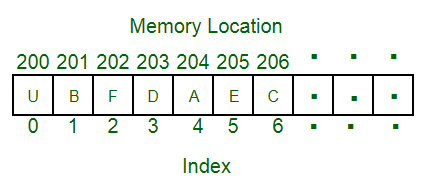Fullsize Image / Reference
Static data structure, referenced via indices `intArray[i][j] = ...` Fixed amount of data (cannot grow), easy access, mathematical operations
ListFullsize Image / Reference
Objects connected by (usually) bidirectional links `list.add(i, obj)`
`list.get(i)`
`list.iterator()`
Dynamic, linear structures, where objects can be added at start, end, or middle
Stack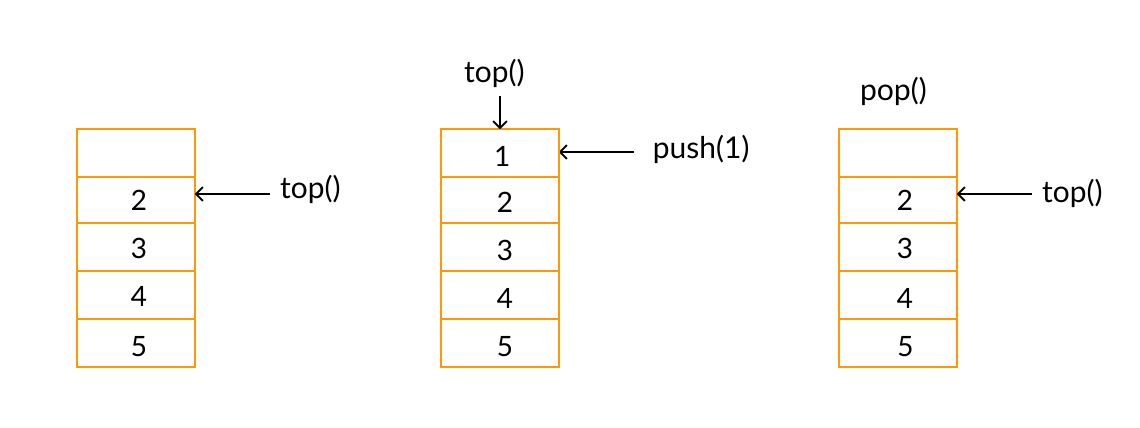Fullsize Image / Reference
List where objects can only added and removed on top `stack.top()`
`stack.push(obj)`
`stack.pop()`
Task management using LIFO (last in, first out) principle
QueueFullsize Image / Reference
List where objects added on one end, and taken out at the other `queue.enqueue(obj)`
`queue.dequeue()`
Manage tasks according to waiting line (FIFO, first in, first out) principle
Map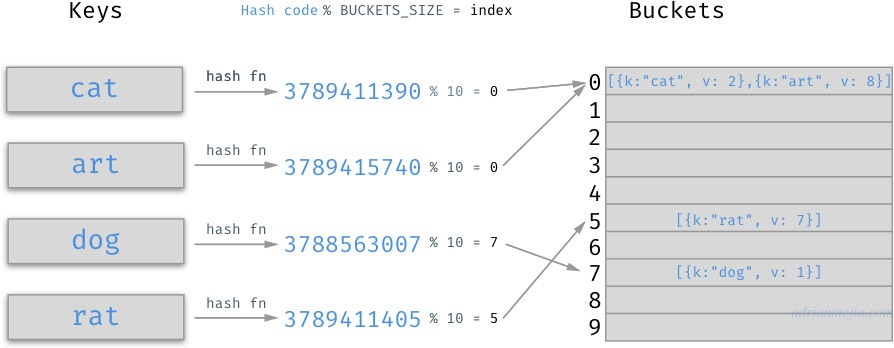Fullsize Image / Reference
Implements a function `key => value (object)` \ `map.set(i, obj)`
`map.get(i)`
Manage a limited number of objects with a huge range of possible keys, with quick access
Set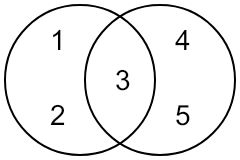Fullsize Image / Reference
Contains an object at max once `set.add(obj)`
`set.remove(obj)`
`set.contains(obj)`
Manage structures where duplicates need to be removed

# Big-O Notation

The big-O notation "O(...)" is a way of expressing how well a data structure performs with growing numbers of elements.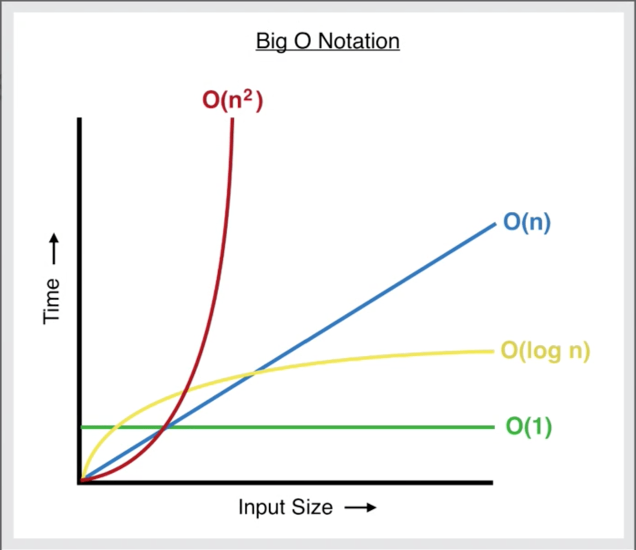• O(1): Effort is (more or less) constant, regardless of the number of elements. Great for large number of elements. May be slow (in absolute time), though. So, suitability for small number of elements need to be checked in each case.
• O(log(n)): 2nd-best option for large numbers - the larger the number, the closer to constant effort.
• O(n): suited for small numbers, less so for large numbers.
• O(n2) (and all higher potences or exponential orders ...): avoid for large numbers!

## Effort For Typical Operations In The Various Data Structures

Assumption in the following table: The element that is inserted / accessed / searched for / deleted is located "somewhere in the middle" of the structure.

Data Structure Insert Access Search Delete Comments
Array O(n) O(1) O(log(n)) O(n) Assumption for search effort: binary search (interval splitting), linear search is O(n)
List O(n) O(n) O(n) O(n)
Stack O(1) O(1) - O(1) insert / access / delete refers to the top-most element only, search is not supported
Queue O(1) O(1) - O(1) insert refers leftmost element only, access / delete to rightmost only, search is not supported
HashMap O(1) O(1) O(1) O(1) Implemented using hash array
TreeMap O(log(n)) O(log(n)) O(log(n)) O(log(n)) Implemented using binary search tree
Set O(1) O(1) O(1) O(1) Assumption: Implemented using HashMap